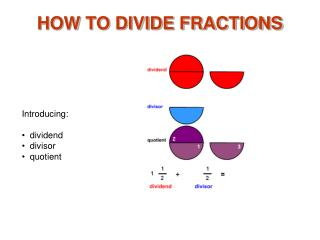DownloadDownload PresentationHOW TO DIVIDE FRACTIONS

# HOW TO DIVIDE FRACTIONS

Download Presentation## HOW TO DIVIDE FRACTIONS

- - - - - - - - - - - - - - - - - - - - - - - - - - - E N D - - - - - - - - - - - - - - - - - - - - - - - - - - -
##### Presentation Transcript

1. HOW TO DIVIDE FRACTIONS • Introducing: • dividend • divisor • quotient

2. Divide Fractions 1 Division is a form of subtraction. This picture shows that the divisor1/2 can be subtracted 3 times from the dividend 1 1/2 . A quotient 3 tells us how many times the divisor can be subtracted from the dividend. By looking at the picture you can see that the divisor fits into the dividend 3 times

3. Divide Fractions 2 To calculate the quotient, first write the dividend and divisor in fraction form. Then multiply 3/2 by the inverse of 1/2 . This gives a quotient of 3/2 x 2/1 or 3.

4. Divide Fractions 3 This picture shows that 1 3/4 can be subtracted from 5 1/4 three times. It also shows how the quotient is calculated.

5. Divide Fractions 4 The same example with number lines shows that the divisor 1 3/4 fits into the dividend 5 1/4 three times. Notice each numbered unit in the quotient is a divisor length and the quotient length is the same as the dividend.

6. Divide Fractions 5 The divisorhas been decreased to 1 1/4. Notice the quotient is increased to 41/5. As the divisor decreases, the quotient increases. Notice that the quotient is straight down from the dividend. That is because we are asking for the number of divisor lengths that can fit into the dividend.

7. Divide Fractions 6 The divisor has been decreased to 1. Notice the quotient is increased to 51/4. Dividing by 1 gives a quotient equal to the dividend.

8. Divide Fractions 7 When the divisor is less than 1, the quotient is larger than the dividend. Notice that there are 7 divisor lengths in the dividend.

9. Divide Fractions 8 Decreasing the divisor to 1/2 increases the quotient to 10 1/2. Notice that there are 10 full divisor lengths that can fit into 5 1/4 plus 1/2 another divisor length.

10. Divide Fractions 9 When the divisor is smaller than the dividend, the quotient is more than 1. Here, it is easy to see that 11/2 divisor lengths fit into the dividend.

11. Divide Fractions 10 Another example where the divisor smaller than the dividend.

12. Divide Fractions 11 This image uses circles to show that if the divisor is the same size as the dividend, the quotient is 1.

13. Divide Fractions 12 When the divisor is larger than the dividend, the quotient is less than 1. The quotient shows that 2 out of 3 divisor circles fit into the dividend.

14. Divide Fractions 13 Another example where the divisor is larger than the dividend. Of course, the quotient can be calculated by multiplying by the reciprocal as is shown. The picture shows the dividend and divisor circles sectioned into 12 parts (the common denominator of 4 and 3). Doing this helps us to see that 21 of the divisor parts fit into the dividend.

15. Divide Fractions 14 What is the quotient of 3 3/4 and 11/2?

16. Divide Fractions 15 Notice that two complete 1 1/2 divisors fit into the dividend. Then only three of the 6 divisor sections will fit into the dividend for 2 1/2 divisors that will fit into the dividend. The image shows how the quotient of 3 3/4 and 11/2 is calculated.